# Magnetics Effects of a Current Carrying Conductor – Straight Wire

Magnetic Field Pattern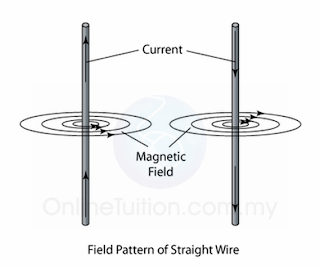(Figure (a))
1. The magnetic field generated by a straight wire are concentric circles around the wire as shown in figure (a) above.
2. Take notes that when the direction of the current is reversed, the direction of the magnetic field line is also reversed.
3. The direction of the magnetic field line can be determined by the Maxwell’s Screw Rule or the Right Hand Grip Rule.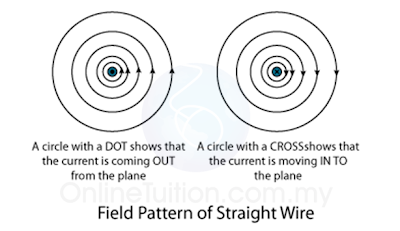(Figure (b): The plan view of the magnetic field generated by a straight wire)
1. Sometime, the magnetic field pattern may be given in plan view, as shown in figure (b).
2. In plan view, a dot in the wire shows the current coming out from the plane whereas a cross in the wire shows the current moving into the plane.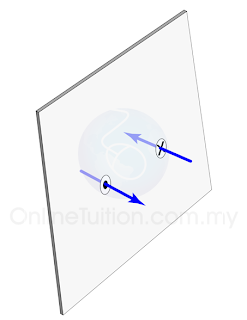(Figure (c): A dot indicates the current move out from a plane whereas a cross indicates the current move into the plane)

Direction of the Magnetic Field

The direction of the magnetic field formed by a current carrying straight wire can be determined by the

1. Right Hand Grip Rule or the
2. Maxwell Screw Rule.

Right Hand Grip Rule
Grip the wire with the right hand, with the thumb pointing along the direction of the current. The other fingers give the direction of the magnetic field around the wire. This is illustrated in the figure below.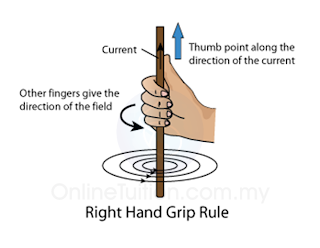(Figure (d))

The Maxwell’s Screw Rules
The Maxwell Screw Rules sometime is also called the Maxwell’s Corkscrew Rule. Imagine a right handed screw being turn so that it bores its way in the direction of the current in the wire. The direction of rotation gives the direction of the magnetic field.(Figure (e))

Strength of the Magnetic Field

1. The strength of the magnetic field form by a current carrying conductor depends on the magnitude of the current.
2. A stronger current will produce a stronger magnetic field around the wire as shown in Figure (f) below.(Figure (f))
3. The strength of the field decreases out as you move further out. This is illustrated in figure (g) below. Thus, you must be very careful when you are asked to draw the magnetic field in your exam.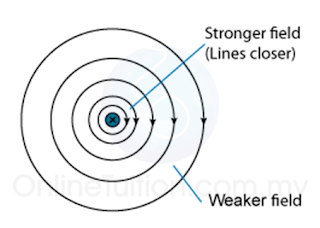(Figure (g)
4. The distance of the field lines must increase as it is further out form the wire.

## External Resources

XXX

### 2 thoughts on “Magnetics Effects of a Current Carrying Conductor – Straight Wire”

1.this site is very use full 4me

2.can you make all these Physic's notes in two modules , form 4 and 5, please?